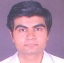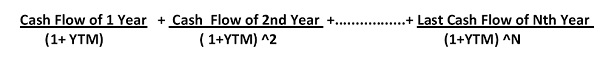# Yield To Maturity (YTM) — An Important Investment Tool

Vikalp SainiDefinition:

The “Yield To Maturity” or “Redemption Yield” of the bonds (such as Corporate Bond or T- Bills) is the internal rate of return which investor is going to earn assuming that investor purchase the bond paying price equal to market price and hold the bond until maturity and issuer paid the principal and interest on the bond as per schedule.

For the Investment analysis and comparison, Yield To Maturity helps the Investors to compare the bonds with different maturities.

Factors Required to Calculate YTM:

1. Par Value
2. Current Market Value
3. Coupon Interest Rate
4. Time To Maturity

Before going forward, let us understand above terminology

1. Par Value also called as “FACE VALUE” is the amount bond holder will receives when bond gets matures.
2. Current Market Value is value of bond at which bond is trading in the market.
3. Coupon Rate is the rate of interest paid on the bond, it is mostly expressed in percentage terms. Interest payment can take place in quarterly, semi-annually or annually as per bond terms.
4. Time To Maturity (is also Denoted as “N” in YTM calculation) is time remaining to bond get mature.

Description:

YTM is the rate at which Cash Flow of the bond is equal to Market Price. YTM can be calculated at any point of time of Bond period.

YTM discounts the future cash flows of the bond and present value of all the cash flow of the Bond = Market Value

FORMULA:

Bond Price/ Market Price =Here,

Cash Flow will be Coupon Rate paid on the Bond i.e Interest Rate and Last Cash Flow comprises of Interest plus Principal amount, depending upon the terms and condition of Bond. There can be possibility that Cash Flow contain part of principal amount and Interest on Reducing Balance of Principal.

Let us understand calculation of YTM with an example

Example: A Reliance Industries 3 years Bond is Paying Coupon Rate of 5% have face value of Rs 100 is having market price of Rs 90. Calculate YTM of the Bond.

Answer: Rs 5 / ( 1+YTM) + 5 ( 1+ YTMA2) + 105 ( YTMA3) = Rs 90

YTM is 8.95 % p.a.

Relationship Between YTM and Coupon Rate

1) If YTM = Coupon Rate, Bond will be price at Par.

2) If YTM < Coupon Rate, Bond will be priced at premium.

3) If YTM > Coupon Rate, Bond will be priced at discount. Conclusion:

Thus, Yield To Maturity is very important tool for investor who want to invest in Debt securities. Investors should calculate YTM of Bond before buying them from secondary market as it is very important investment tool to understand that whether Bond is trading at premium, discount or at par.

October 2020
M T W T F S S
1234
567891011
12131415161718
19202122232425
262728293031